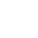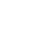LV. 49
GP 7k^^^^^^^^^^^^^^^^^^^^^^^^^^^^^^^^^^^^^^^

https://forum.gamer.com.tw/C.php?bsn=40128&snA=325&tnum=1

^^^^^^^^^^^^^^^^^^^^^^^^^^^^^^^^^^^^^

^^^^^^^^^^^^^^^^^^^^^^^^^^^^^^

^^^^^^^^^^^^^^^^^^^^^^^^^^^

face基於日前微軟官方表示 Internet Explorer 不再支援新的網路標準，可能無法使用新的應用程式來呈現網站內容，在瀏覽器支援度及網站安全性的雙重考量下，為了讓巴友們有更好的使用體驗，巴哈姆特即將於 2019年9月2日 停止支援 Internet Explorer 瀏覽器的頁面呈現和功能。

。Google Chrome（推薦）
。Mozilla Firefox
。Microsoft Edge（Windows10以上的作業系統版本才可使用）

face我們了解您不想看到廣告的心情⋯ 若您願意支持巴哈姆特永續經營，請將 gamer.com.tw 加入廣告阻擋工具的白名單中，謝謝 !【教學】